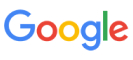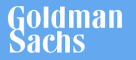New update is available. Click here to update.
Last Updated: 27 Feb, 2021

# Ninja And The Game Of Words

Moderate+5 more companies

## Problem statement#### For Example:

``````‘STR’ = “i am a Ninja”, ‘N’ = 3 and ‘WORDS[]’ = [“Ninja”,”a”,”am”]. Then the output should be [1,3,1]. Because the occurrence of “Ninja” in ‘STR’ is 1 and the occurrence of “a” in ‘STR’ is 3.Similarly occurrence of “am” is 1.
``````

#### Note:

``````The output should be in the same order as given in ‘WORDS’.
``````

#### Can you help Ninja to generate all valid strings from ‘STR’ by minimum removals?

##### Input Format:
``````The first line of input contains an integer  'T' which denotes the number of test cases.

The first line of each test case contains an integer ‘N’.

The second line contains a string ‘STR’.

The next ‘N’ lines contain a string representing words.
``````
##### Output Format :
``````For each test case, return the frequency of all the words given in ‘WORDS’ Array/List

Print the output for each test case in a separate line.
``````
##### Note:
``````You don't need to print anything; it has already been taken care of. Just implement the given function.
``````
##### Constraints:
``````1 <= ‘T’ <= 100
1 <= |STR| <= 4000
1<= N <= 4000
1<= |WORDS[i]| <= 4000

Where  |'STR'| denotes the length of the given string and ‘|WORDS[i]|’ denotes the length of the string word.

Time limit: 1 sec
``````## Approaches

### 01 ApproachThe idea behind this approach is to one by one check all the words in ‘WORDS’ if they are present in ‘STR’ or NOT. If present then increases the count of that word.

Here is the complete algorithm:

• Make an array/list ‘ANSWER’ to store the count of occurrence of each word. Iterate the ‘WORDS’ and for each word ‘WORD[i]’ at index ‘i’ in the ‘WORDS’ do the following:
• Inetialize a variabe ‘COUNT’ = 0.
• Iterate the ‘STR’ and for each index ‘j’ do the following:
• If the substring of length from ‘j’ to ‘j + lengthof(WORD[i])’ is equal to the ‘WORD[i]’ then increase the ‘count’ by ‘1’.
• Insert the value of ‘COUNT’ in the ‘ANSWER’.